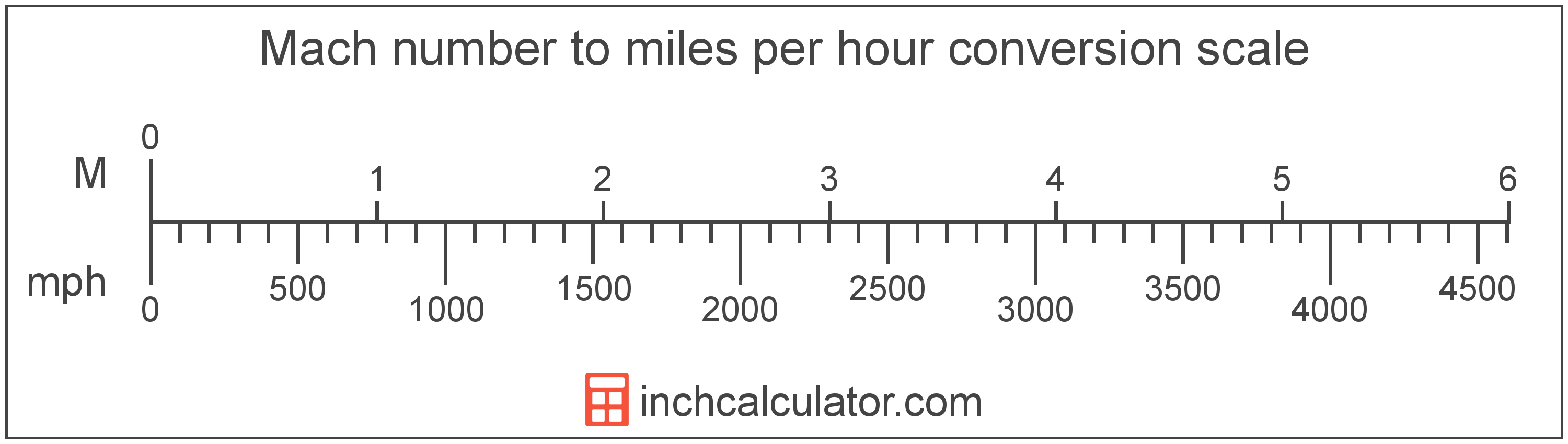# Miles per Hour to Mach Number Converter

Enter the speed in miles per hour below to get the value converted to Mach number.

Results in Mach Number:1 mph = Mach 0.001303

Do you want to convert Mach number to miles per hour?

## How to Convert Miles per Hour to Mach Number

To convert a mile per hour measurement to a Mach measurement, multiply the speed by the conversion ratio. One mile per hour is equal to Mach 0.001303, so use this simple formula to convert:

Mach number = miles per hour × 0.001303

The Mach number is equal to the speed in miles per hour multiplied by 0.001303.

For example, here's how to convert 5 miles per hour to Mach number using the formula above.
5 mph = (5 × 0.001303) = Mach 0.006517Miles per hour and Mach number are both units used to measure speed. Keep reading to learn more about each unit of measure.

## What Are Miles per Hour?

Miles per hour are a measurement of speed expressing the distance traveled in miles in one hour.

The mile per hour is a US customary and imperial unit of speed. Miles per hour can be abbreviated as mph, and are also sometimes abbreviated as mi/h or MPH. For example, 1 mile per hour can be written as 1 mph, 1 mi/h, or 1 MPH.

Miles per hour can be expressed using the formula:
vmph = dmi / thr

The velocity in miles per hour is equal to the distance in miles divided by time in hours.

## What is Mach Number?

Mach, sometimes also referred to as a Mach number, is the ratio of the speed of an object to the speed of sound.

Mach can be abbreviated as M, and is also sometimes abbreviated as Ma. For example, Mach 1 can be written as 1 M or 1 Ma.

Although Mach can be abbreviated M or Ma, the Mach number is typically expressed with the number after the unit name. For example, the expression Mach 3 is preferred rather than 3 Machs or 3 M.

The speed is classified as either subsonic, transonic, supersonic, or hypersonic depending on the Mach number.

Speed classifications for various mach numbers.
Speed Classification Mach Number
Subsonic Mach < 1.0
Transonic Mach = 1.0
Supersonic Mach > 1.0
Hypersonic Mach > 5.0

## Mile per Hour to Mach Conversion Table

Mach numbers converted to Mach number
Miles Per Hour Mach Number
191.82 mph Mach 0.25
383.63 mph Mach 0.5
575.45 mph Mach 0.75
767.27 mph Mach 1
1,151 mph Mach 1.5
1,535 mph Mach 2
1,918 mph Mach 2.5
2,302 mph Mach 3
2,685 mph Mach 3.5
3,069 mph Mach 4
3,453 mph Mach 4.5
3,836 mph Mach 5
4,220 mph Mach 5.5
4,604 mph Mach 6
4,987 mph Mach 6.5
5,371 mph Mach 7
5,755 mph Mach 7.5
6,138 mph Mach 8
6,522 mph Mach 8.5
6,905 mph Mach 9
7,289 mph Mach 9.5
7,673 mph Mach 10

## References

1. Wikipedia, Miles per hour, https://en.wikipedia.org/wiki/Miles_per_hour
2. NASA, Mach Number, https://www.grc.nasa.gov/WWW/BGH/mach.html